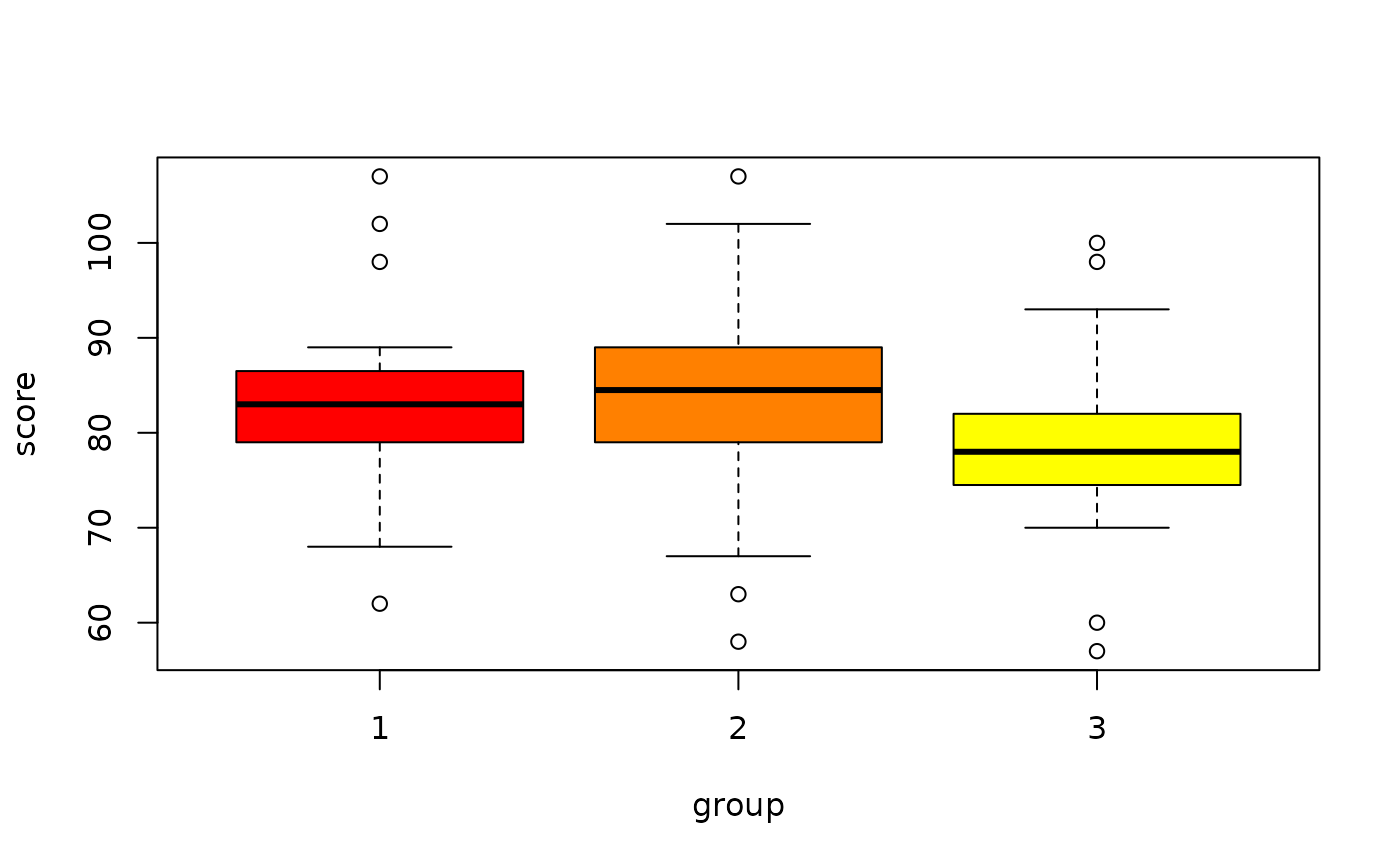Data for Exercise 10.45

Longtail

## Format

A data frame/tibble with 60 observations on three variables

score

a numeric vector

group

a numeric vector with values 1, 2, and 3

ranks

ranks for variable score

## Examples


boxplot(score ~ group, data = Longtail, col = heat.colors(3))kruskal.test(score ~ factor(group), data = Longtail)
#>
#> 	Kruskal-Wallis rank sum test
#>
#> data:  score by factor(group)
#> Kruskal-Wallis chi-squared = 5.5622, df = 2, p-value = 0.06197
#>
anova(lm(score ~ factor(group), data = Longtail))
#> Analysis of Variance Table
#>
#> Response: score
#>               Df Sum Sq Mean Sq F value Pr(>F)
#> factor(group)  2  333.7  166.87  1.3917  0.257
#> Residuals     57 6834.5  119.90